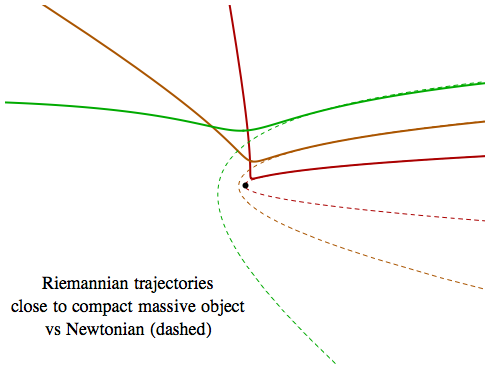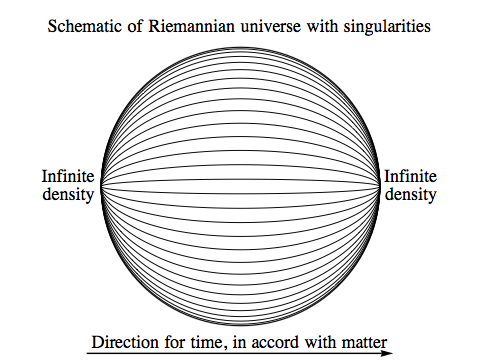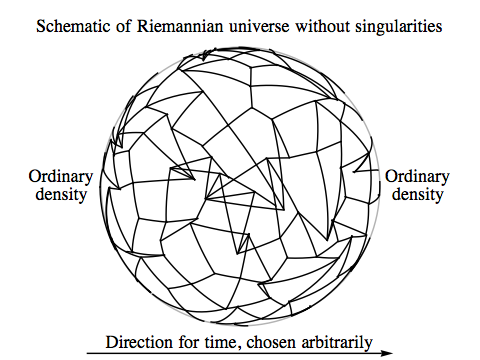# Orthogonal

## Riemannian General Relativity

Orthogonal

### Yes, We Have No Black Holes

How different is the behaviour of gravity in the Riemannian universe from the way it works in our own?

Here’s one possible change: since there is neither a fixed speed of light nor any speed limit on material objects, it would seem that there can be no black holes. If you can travel at arbitrarily high speeds, then surely whatever the escape velocity of a massive body, you could, in principle, achieve that speed.

On the other hand, the kinetic energy of an object does not increase without limit as its velocity grows. As we discussed previously, the kinetic energy approaches the rest mass of the object as its velocity approaches infinity. So what if you are in a gravitational potential well so deep that your potential energy is less than –m, the opposite of your rest mass? At least in Newtonian gravity, the potential energy of an object with mass m at a distance r from a star or planet of mass M is:

U = – G M m / r

which would imply that for r < G M, the potential energy well is too deep for an object to escape, even if it’s travelling at an infinite velocity and has a kinetic energy of m.

The only way to resolve the issue is to apply Einstein’s theory of general relativity, which can be adapted easily to Riemannian four-space. The details are on the extra page for this topic; here we’ll just sketch a few interesting results from Riemannian general relativity, without proof.

The escape velocity from a massive body can become arbitrarily high, but it turns out that the form it takes is exactly what you’d get if you combined Newtonian gravitational potential energy with the Newtonian formula for kinetic energy, which increases without bound as the velocity increases. To be specific, it turns out that for an object in free fall moving radially towards or away from a massive body, the quantity:

E2 = (1 + 2 G M / r) / (1 + v2)

is conserved. This is the Riemannian-general-relativistic version of conservation of energy in a gravitational field. As the object descends and falls faster, or as it climbs away from the massive body and is slowed in its ascent, this quantity remains the same. So in order for an object to have a speed of 0 when its distance from the massive body is infinite — the condition for it to be moving at escape velocity — what is required is for E2 to equal 1. In other words:

(1 + 2 G M / r) / (1 + vesc(r)2) = 1
vesc(r)2 = 2 G M / r
(1/2) m vesc(r)2G M m / r = 0

The last line states that the sum of the Newtonian version of kinetic energy and the Newtonian version of the potential energy is zero. The same final result is actually true in our own universe as well — but in our universe v must be less than 1, so at r = 2 G M escape is impossible and you’ve crossed the event horizon of a black hole. In the Riemannian universe, as our first intuition suggested, the fact that there’s no limit on v means that gravity can never grow too strong to overcome.Although there are no Riemannian black holes, the curved four-space around a sufficiently compact massive body can still have some exotic effects. The first is time dilation, which works in the opposite way to gravitational time dilation in our universe. In the Riemannian universe, if you spend time close to a massive object then rejoin a companion who kept their distance, more time will have passed for you than for your companion.

Another effect is the way curvature modifies centrifugal force. In our universe, if you get too close to a black hole, centrifugal force effectively goes into reverse and helps push you into the hole. In the Riemannian universe, centrifugal force always repels, and it can be even stronger than its Newtonian counterpart. As a result, if you pass too close to a compact, massive object then instead of swinging around it in a Newtonian hyperbolic orbit, you will be deflected away from it.

Because of the stronger centrifugal force, there is a limit to how fast a planet can be moving in its orbit — no matter how close it is to the star it’s orbiting, or how massive the star. No circular orbit can exist with a speed greater than 1/√2; if the planet is moving faster than that, centrifugal force will overwhelm gravitational attraction.

### No Gravitational Waves

The equation that governs gravitational waves in the Riemannian universe is potentially subject to the same kind of problem we saw with light waves, where waves of too high a frequency in one dimension would undergo exponential growth in another. But for gravitational waves the situation is even more dire, because it would happen with waves of any frequency at all. There is no maximum frequency that needs to be exceeded.

As a result of this, the mechanism that prevents the exponential growth of light waves eliminates gravitational waves completely. In our universe, two massive objects that are orbiting each other very close together will radiate away energy in gravitational waves. This causes them to spiral inwards, and eventually collide. In the Riemannian universe, any energy that went into creating gravitational waves would have the opposite effect, causing the orbiting bodies to move apart ... but in fact, this won’t happen, because no gravitational radiation is possible.

### ... and Probably No Big BangIn Lorentzian physics, if you follow the history of most simple models of the universe back in time, you end up at a moment when everything was infinitely dense and the classical laws of general relativity must fail: the Big Bang.

In Riemannian physics, this kind of model of the universe is possible, but it’s very artificial and contrived. It requires you to assume that the universe is full of matter whose world lines are all pointing in roughly the same direction. This might be a natural assumption in the Lorentzian universe, where world lines are confined to lie within a cone of timelike directions, but in the Riemannian universe they can be oriented along any direction in four-space.

If we make the more natural assumption, in the Riemannian case, that on a cosmic scale the world lines of matter exhibit no preference at all for a particular direction, then if we choose a direction and call it “time”, the average density of matter as we go back in time need not change at all. The universe can still shrink to zero volume, but the particular event where this happens is just an artifact of our choice of a time direction — just as the shrinking of circles of latitude to zero at the north and south pole is an artifact of our choice of coordinates.

For the sake of simplicity, in the images on the right these models are both drawn on the surface of a sphere, but the four-space will only really be positively curved in all directions if there is a sufficiently large, positive cosmological constant — which in the Riemannian case is equivalent to a large negative vacuum energy. Without a cosmological constant, the first model will have positive curvature in the “time” direction and negative curvature in the other three. This means that a cloud of free-falling particles will be squeezed into a smaller volume if their world lines are more or less aligned with all the other matter in the universe, but if they are travelling at infinite speed relative to that background matter, they will instead become more spread out.

The second model, without a cosmological constant, will have negative curvature in all directions.

There is extra material on this topic for readers who don’t mind a slightly higher level of mathematics.Orthogonal / Riemannian General Relativity / created Wednesday, 6 April 2011